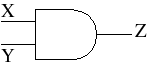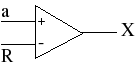## Stochastic Computing

### The Problem with Multiplication

Consider the sum of two numbers :

0.1234 + 0.5555 = 0.6789

We can do this in our heads because only one addition is needed per digit. Working our way from the the least significant digit to the most significant we get

4+5 = 9
3+5 = 8
2+5 = 7
1+5 = 6
0+0 = 0

If the sum of any two digits is greater than 9 then we need to mentally carry over an extra 1 to the sum of the next greater pair of digits. This is not at all difficult. Sumation in general is a fairly simple task, and its simplicity carries over to the digital circuitry in the calculators and personal computers we use today. Multiplication, on the other hand, is much more complicated:

(0.1234)(0.5555) = ?

I'm not going to try do this in my head, it's simply too painful. In an emergency I'm confident I could crunch the numbers and get a result of 0.0685487, but my first reflex is to look for the nearest calculator. Before the 1970s when inexpensive calculators became available, engineers often used slide rules for difficult computations like this. On my trusty Keuffel and Esser Decitrig, for example, which is a slide rule I haven't routinely used since 1974, I get a result of

(0.1234)(0.5555) = 0.0686

Trying to squeeze out the last 6 is difficult, even on a high-precision instrument like the Decitrig. Could it possibly be 0.0685 or 0.0687? It's hard to be sure from where the cursor sits on the scale. For most engineering applications, however, a result of 0.0686 plus or minus 0.0001 is plenty accurate.

In the digital age we still need to multiply, but instead of straining our brain or our eyes we let digital logic take over. Such a feat requires a lot of transistors. Consider, for example, the Intel 8088 microprocessor that graced the motherboards of the first IBM personal computers. It could add long numbers together with ease, but multiplication was another story. Multiplication took a long time because the chip had to more or less do what we used to do in Mrs. Schmidt's 3rd grade math class: break the multiplication down into a long series of simple products and additions. To speed up the process, Intel created the 8087 math coprocessor, an entire chip dedicated to the task of multiplying two numbers together. Even the mighty 32-bit 80386 chip needed an external 80387 math coprocessor. It wasn't until the 80486 that all those multiplying transistors could be squeezed onto the main chip. To this day modern digital signal processors dedicate huge amounts of circuitry to multiplication.

So here's a tantalizing thought: what if we could multiply 2 numbers together using just a single AND gate?The AND gate produces an output Z=1 only if its inputs are X=1 and Y=1. If either input is zero then the output is zero. This is not a very complicated device. It's about as simple as they get. So how can we possibly use such a small circuit to multiply numbers like 0.1234 and 0.5555?

### A Strange Way to Represent Numbers

What if we were to describe each number by a probability? Here's how it would work. Consider, for example, the product (0.5)(0.25). For the first input of 0.5 we flip a coin. If it comes up heads then we set the upper input of the AND gate to X=1. If it's tails then X=0. There is a 50% chance of X=1. In other words the probability of X=1 is 0.5. As a mathematical formula we express this as

P{X=1} = 0.5

The input X can only be zero or one. We won't know which of these values it will have until the coin is tossed, but because the probability of X=1 is 0.5, then X represents the input 0.5.

For the second input of 0.25, let's put one white marble and three black marbles into a hat and pick one without looking. If the marble picked is white then Y=1. If it is black then Y=0. There is a 1 out of 4 chance of the marble being white, or in mathematical terms

P{Y=1} = 0.25

Thus Y represents the number 0.25.

To represent a number by a probability is a very strange concept, but here's why it warrants further investigation. Because of the AND gate, the output Z is a one only if both inputs are ones. Since the chance of X being one is 1/2 and the chance of Y being one is 1/4, then the chance of Z being one is (1/2)(1/4) = 1/8, or 0.125. Like its inputs, the AND gate output can only be a one or a zero. We don't know which until the coin is tossed and the marble is picked, but the probability of it being a one is precisely 0.125. Thus Z represents the number 0.125 the same way that X represents 0.5 and Y represents 0.25. It is equal to the product (0.5)(0.25) expressed as a probability. So by using this strange, "stochastic" representation of numbers we get an output that equals the product of the two inputs.

All of this works because of a fundamental priniciple: the probability of two statistically independent events is equal to the probability of the first event times the probability of the second. We represent any two arbitrary numbers a and b, each falling within the range of zero to one, by the inputs X and Y.

We start by making P{X=1} = a and P{Y=1} = b. Because of the AND gate we get

P{Z=1} = P{X=1 and Y=1}

and because the two events X=1 and Y=1 are independent this results in

P{X=1 and Y=1} = ( P{X=1} )( P{Y=1} )

Combining these two equations proves that we get the desired result

P{Z=1} = (a)(b)

Any two numbers between zero and one can be multiplied by the AND gate. If we could somehow make the probability of X=1 equal to 0.1234, and the probability of Y=1 equal to 0.5555, for example, then P{Z=1} is precisely 0.0685487. To generate these inputs we need something more practical than coins or marbles. One such system consists of a comparator and a random number generator.Here a is a number between zero and one that we wish to multiply. R is a randomly generated number with the same number of bits as a. It is "uniformly distributed," meaning that any number between zero and one is equally likely. The chance of it being equal to 0.781 is the same as 0.003 or any other number between zero and one. (This is usually accomplished in practical circuits by simply making all of the bits that make up R statistically independent and each equally likely to be a one or a zero. In other words, the individual bits that make up R are completely random.)

The comparator produces a one if the input a is greater than the random number R. Otherwise it outputs a zero. If a=0.75, for example, then there is a 75% chance that it will be greater than R and thus P{X=1} = 0.75. More generally, the circuit ensures that

P{X=1} = P{a>R} = a

The comparator is performing the task of a binary-to-stochastic code converter because it converts a deterministic number represented by bits into a stochastic number represented by a probability.

The flip of a coin has no influence on the color of a marble. Similarly, a separate, statistically independent source of random numbers and another comparator are needed for b. This makes X and Y statistically independent. Any statistical correlation between them would inevitably corrupt the result.

### There's No Free Lunch

There's a catch to all this. Somehow we need to convert the stochastic output Z into the deterministic value c = (a)(b). Even though P{Z=1} = c is a precise equation, we can only estimate the value of c based on our knowledge of Z. If we were to flip the coin and draw a marble 10 times in a row, for example, we might get, say, one occurance of Z=1 and 9 occurances of Z=0. From this we would estimate that c = 1/10 = 0.1. This is fairly close to the correct answer but much worse than what we would get with a slide rule. If we repeat the process until we have 100 values of Z then we could get 12 or so occurances of Z=1 and estimate the result to be c = 12/100 = 0.12. A result of 0.11 or 0.13 might also easily occur. There is even a slight chance that through an evil twist of fate the estimate is c = 0.0 or c = 1.0. It is, after all, theoretically possible to win the million-dollar state lottery. Our estimate gets more accurate, and the chance of a bizarre answer becomes less likely, when we use more samples.

To get a really accurate estimate we need a lot of samples, and that takes a long time. Stochastic multiplication thus greatly reduces the size of the circuit, but also greatly increases the time required to get an accurate answer. If the circuit has a clock speed measured in MHz and the input signal contains frequencies measured in Hz, then stochastic computing has some real potential. This is often the case for AC power test equipment, for example, or for the measurement of physical quantities like temperature and pressure.

Another potential application is in neural networks, where the accuracy of a single multiplication is often not as important as the cumulative effect of thousands of multiplications being performed simultaneously. A biological neuron, after all, is not a terribly accurate device, but if millions of them are combined into the complex structure that we call the human brain, then you get the most capable cognitive system on the planet! Even if we can't multiply long numbers in our heads.